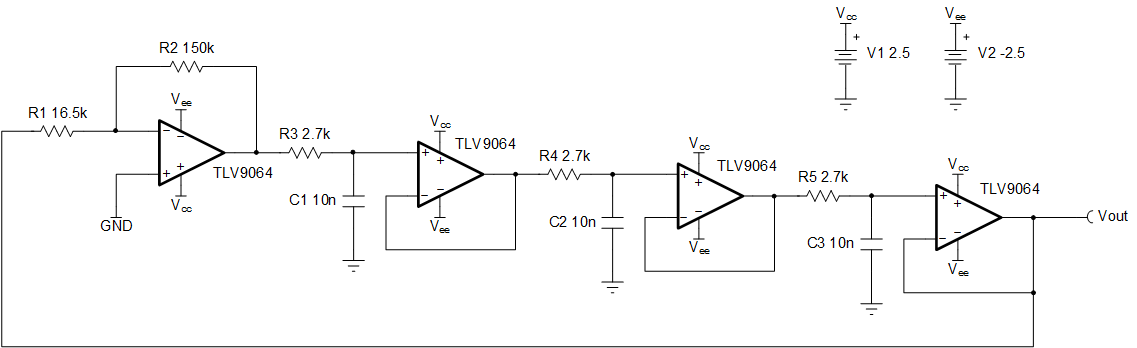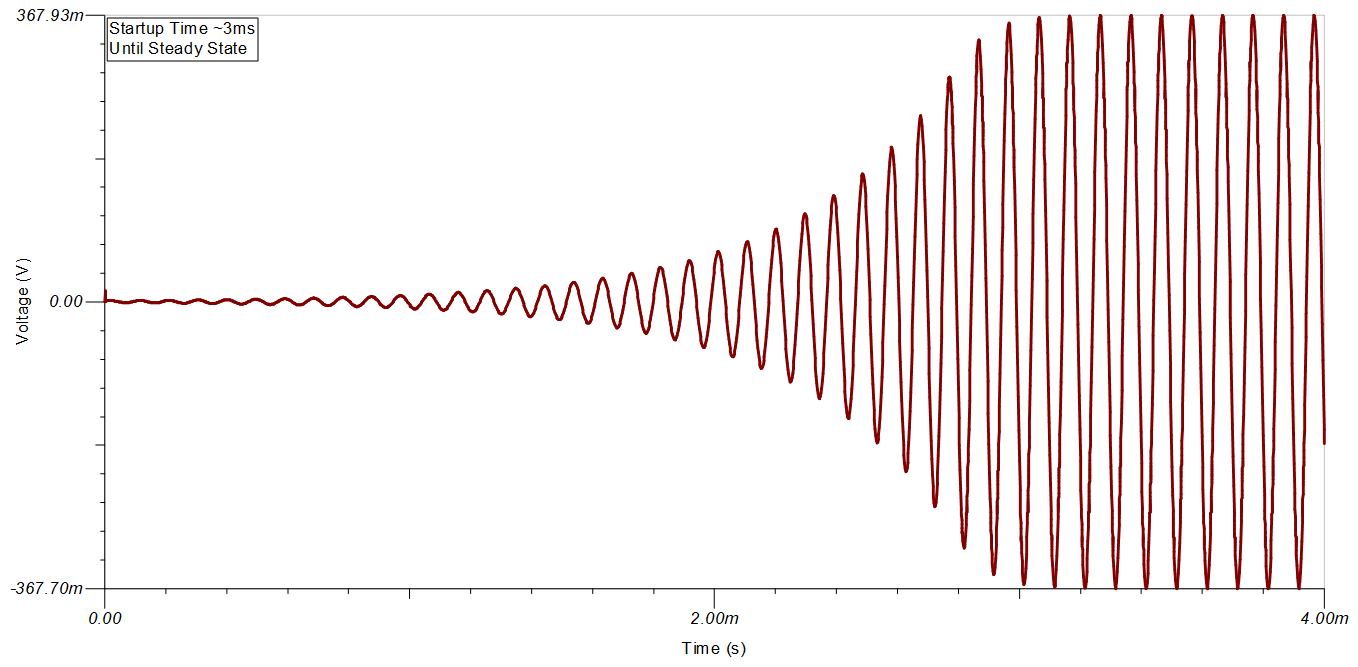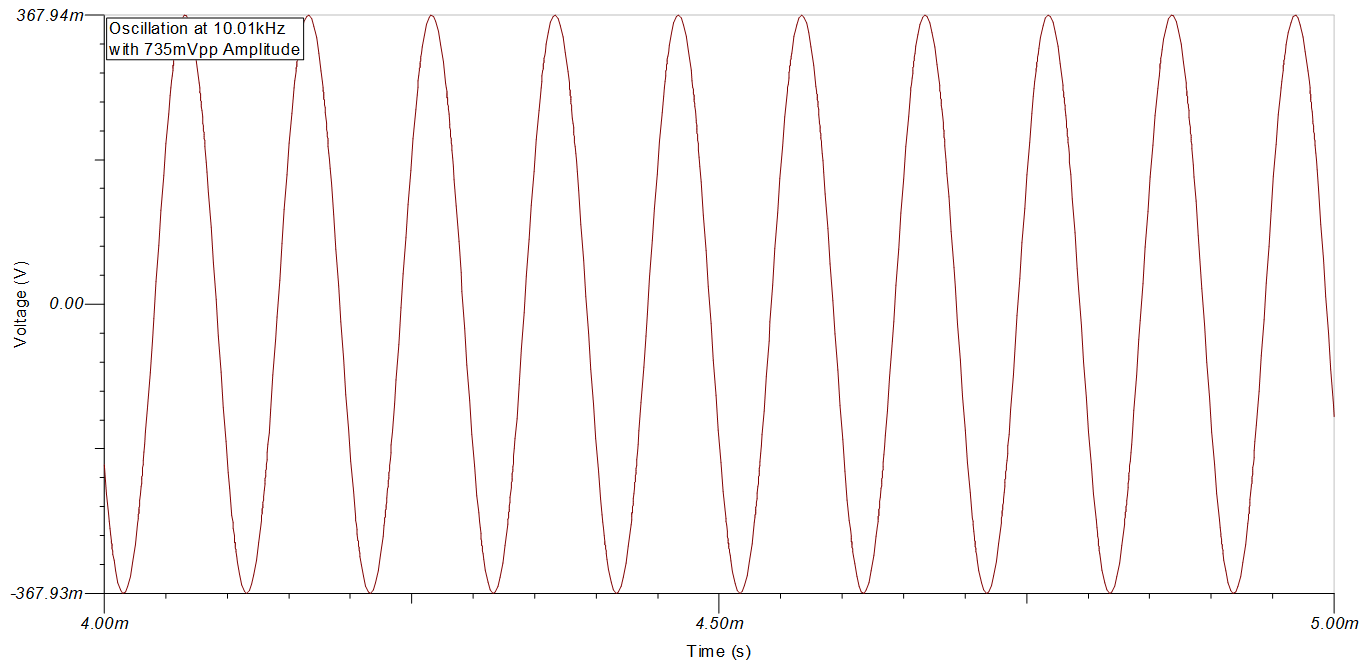SLOA278 July   2021

Design Goals

AC Specifications Supply
AC Gain foscillation Vcc Vee
8V/V 10kHz 2.5V –2.5V

Design Description

This circuit uses a quad channel op amp with ±2.5-V supplies to generate a 10kHz, low-distortion sine wave. The amplifiers buffer each RC filter stage, which yields a low-distortion output.Design Notes

1. Using excessively large feedback resistors, R1 and R2, can lead to a shift in oscillation frequency, and an increase in noise and distortion.
2. The first stage resistors, R1 and R2, must be selected to provide a sufficiently large gain. Otherwise, oscillations at the output will dampen. However, an excessively large gain at the first stage will lead to higher output distortion and a decreased frequency of oscillation.
4. At higher frequencies (> 10 kHz), the phase delay of the amplifier becomes significant. The result will be a frequency of oscillation that is lower than calculated or expected. Thus, some margin must be included when selecting values for the loading elements of the first, second, and third stages (R3, R4, R5, C1, C2, and C3) for higher-frequency designs to ensure the desired oscillation frequency is achieved.
5. Choose an amplifier with at least 100 times the required gain bandwidth product. This will ensure the actual and calculated oscillation frequencies match.
6. For more precise control of the oscillation frequency, use passive components with lower tolerances.

Design Steps

For a classical feedback system, oscillation occurs when the product of the open loop gain, AOL, and the feedback factor, β, is equal to –1, or 1 at 180°. Therefore, each RC stage in the design must contribute 60° of phase shift. Since each stage is isolated by a buffer, the feedback factor, β, of the first stage must have a magnitude of (1/2)3. Therefore the gain (1/β) must be at least 8V/V.

1. Select the first stage feedback resistors for the gain necessary to maintain oscillation.
$\mathrm{Gain}=\frac{{R}_{2}}{{R}_{1}}\ge 8\frac{V}{V}$
2. Calculate components R3, R4, R5, C1, C2, and C3 to set the oscillation frequency. Select C1, C2, and C3 as 10nF.
${f}_{\mathrm{oscillation}}=\frac{\mathrm{tan}\left(60°\right)}{2\pi ×R×C}=10kHz$
${C}_{1,2,3}=10nF \left(Standard Values\right)$
${R}_{3,4,5}=\frac{\mathrm{tan}\left(60°\right)}{2\pi ×C×{f}_{\mathrm{oscillation}}}=\frac{1.73}{2\pi ×10nF×10kHz}=2757\Omega \approx 2.7k\Omega \left(Standard Values\right)$
3. Ensure the selected op amp has the bandwidth to oscillate at the desired frequency.
${f}_{\mathrm{oscillation}}\ll \frac{\mathrm{GBW}}{\mathrm{Gain}}=\frac{\mathrm{GBW}}{\left(\frac{{R}_{2}}{{R}_{1}}\right)+1}$
$10kHz\ll \frac{10MHz}{\left(\frac{150k\Omega }{16.5k\Omega }\right)+1}\cong 991kHz$
4. Ensure the selected op amp has the slew rate necessary to oscillate at the desired frequency. Use the full power bandwidth equation to calculate the necessary slew rate and ensure it is less than the slew rate of the amplifier. While the exact amplitude of oscillation is difficult to predict, you can ensure that our amplifier is fast enough to generate the needed sine wave by ensuring that the output can swing from rail-to-rail.
${\mathrm{SR}}_{\mathrm{req}}<{\mathrm{SR}}_{\mathrm{TLV}9064}$

Design Simulations

The resulting simulations demonstrate a sinusoidal oscillator that reaches steady state after about 3ms to a 10.01-kHz sine wave with a 735-mVpp amplitude.

Transient Simulation ResultsDesign References

1. See Analog Engineer's Circuit Cookbooks for TI's comprehensive circuit library.
2. SPICE Simulation File: SLOC355.
3. TI Precision Labs
4. Sine-Wave Oscillator Application Report
5. Design of Op Amp Sine Wave Generators Application Report

Design Featured Op Amp

TLV9064
Vss 1.8V to 5.5V
VinCM Rail-to-rail
Vout Rail-to-rail
Vos 300µV
Iq 538µA
Ib 0.5pA
UGBW 10MHz
SR 6.5V/µs
#Channels 1, 2, 4
www.ti.com/product/TLV9064

Design Alternate Op Amps

TLV9052 OPA4325
Vss 1.8V to 5.5V 2.2V to 5.5V
VinCM Rail–to–rail Rail–to–rail
Vout Rail–to–rail Rail–to–rail
Vos 330µV 40µV
Iq 330µA 650µA
Ib 2pA 0.2pA
UGBW 5MHz 10MHz
SR 15V/µs 5V/µs
#Channels 2 4
www.ti.com/product/TLV9052 www.ti.com/product/OPA4325